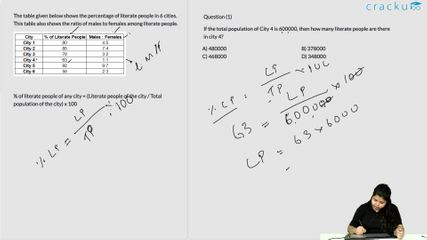### 2017 SSC CGL 09 Aug Shift-2 Question 98

Instructions

The table given below shows the percentage of literate people in 6 cities. This table also shows the ratio of males to females among literate people.

Question 98

# Total population of City 6 is 200000 and the total population of City 2 is 220000. What is the respective ratio of literate males of City 2 and literate females of City 6?

Solution

Total population of City 2 = 220,000

Number of literates of city 2 = 0.85 x 220,000 = 1,87,000

=> Literate males of City 2 = $$\frac{7}{(7+4)}\times1,87,000=119,000$$

Total population of City 6 = 200,000

Number of literates of city 6 = 0.58 x 200,000 = 116,000

=> Literate females of City 6 = $$\frac{3}{(2+3)}\times116,000=69,600$$

=> Required ratio = $$\frac{119,000}{69,600}=\frac{595}{348}$$

=> Ans - (C)

### View Video Solution